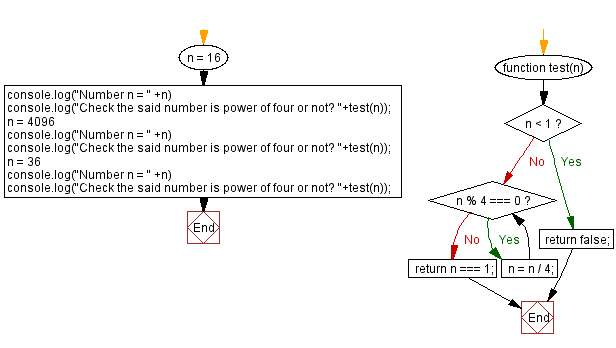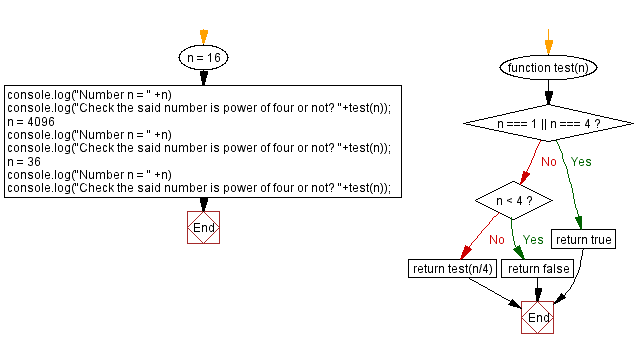# JavaScript: Power of four

## JavaScript Math: Exercise-108 with Solution

Write a JavaScript program to check whether a given integer is a power of four or not.

In arithmetic and algebra, the fourth power of a number n is the result of multiplying four instances of n together. So: n4 = n × n × n × n
Fourth powers are also formed by multiplying a number by its cube. Furthermore, they are squares of squares.
The sequence of fourth powers of integers (also known as biquadrates or tesseractic numbers) is:
0, 1, 16, 81, 256, 625, 1296, 2401, 4096, 6561, 10000, 14641, 20736, 28561, 38416, 50625, 65536, 83521, 104976, 130321, 160000, 194481, 234256, 279841, 331776, 390625, 456976, 531441, 614656, 707281, 810000, ...

Test Data:
(16) -> true
(4096) -> true
(36) -> false

Sample Solution:

HTML Code:

``````<!DOCTYPE html>
<html>
<meta charset="utf-8">
<title>JavaScript program to Power of four or not</title>
<body>

</body>
</html>
```
```

Solution-1

JavaScript Code:

``````function test(n) {
if (n < 1) {
return false;
}

while (n % 4 === 0) {
n = n / 4;
}
return n === 1;
}
n = 16
console.log("Number n = " +n)
console.log("Check the said number is power of four or not? "+test(n));
n = 4096
console.log("Number n = " +n)
console.log("Check the said number is power of four or not? "+test(n));
n = 36
console.log("Number n = " +n)
console.log("Check the said number is power of four or not? "+test(n));
```
```

Sample Output:

```Number n = 16
Check the said number is power of four or not? true
Number n = 4096
Check the said number is power of four or not? true
Number n = 36
Check the said number is power of four or not? false
```

Flowchart:Live Demo:

See the Pen javascript-math-exercise-108 by w3resource (@w3resource) on CodePen.

Solution-2

JavaScript Code:

``````function test(n) {
if(n === 1 || n === 4) return true
if(n < 4) return false

return test(n/4)
}

n = 16
console.log("Number n = " +n)
console.log("Check the said number is power of four or not? "+test(n));
n = 4096
console.log("Number n = " +n)
console.log("Check the said number is power of four or not? "+test(n));
n = 36
console.log("Number n = " +n)
console.log("Check the said number is power of four or not? "+test(n));
```
```

Sample Output:

```Number n = 16
Check the said number is power of four or not? true
Number n = 4096
Check the said number is power of four or not? true
Number n = 36
Check the said number is power of four or not? false
```

Flowchart:Live Demo:

See the Pen javascript-math-exercise-108-1 by w3resource (@w3resource) on CodePen.

Improve this sample solution and post your code through Disqus

What is the difficulty level of this exercise?

Test your Programming skills with w3resource's quiz.

﻿